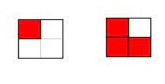# McGraw Hill Math Grade 4 Chapter 7 Lesson 6 Answer Key Identifying Valid Comparisons

Practice the questions of McGraw Hill Math Grade 4 Answer Key PDF Chapter 7 Lesson 6 Identifying Valid Comparisons to secure good marks & knowledge in the exams.

## McGraw-Hill Math Grade 4 Answer Key Chapter 7 Lesson 6 Identifying Valid Comparisons

Solve

Question 1.
Jill paints a board as shown.She says she painted $$\frac{1}{4}$$ of the board yellow. Is she correct? Why or why not?
She is not correct.
Explanation:
$$\frac{1}{4}$$ means the whole has been divided into 4 equal parts.
The board shows 4 parts, but they are not equal parts.

Question 2.
Draw a picture that correctly shows $$\frac{3}{4}$$ > $$\frac{1}{4}$$Explanation:
A square is divided into 4 equal parts
3 parts shaded is greater than one part
as shown in the figure.

Question 3.
$$\frac{2}{4}$$ and $$\frac{4}{8}$$ are equivalent fractions. Explain why the amounts might not be equal.